Homework Help Question & Answers

# 12. The black-scholes OPM is dependent on which five parameters? a. stock price, exercise price, risk...

12. The black-scholes OPM is dependent on which five parameters?

a. stock price, exercise price, risk free rate, beta, and time to maturity

b. stock price, risk free rate, beta, time to maturity, and variance

c. stock price, risk free rate, probability, variance and exervise price

d. stock price, exercise price, risk free rate, variance and time to maturity

13. a six-month call option has an exercise price of US\$45 while the underlying stock currently sells for US\$50. The call option is selling for US\$9.75 and the risk-free rate of return is 7% per annum. What is the value of the put option?

a. 3.20

b. 4.20

c. 6.20

d.cannot be determined

#### Homework Answers

Answer #1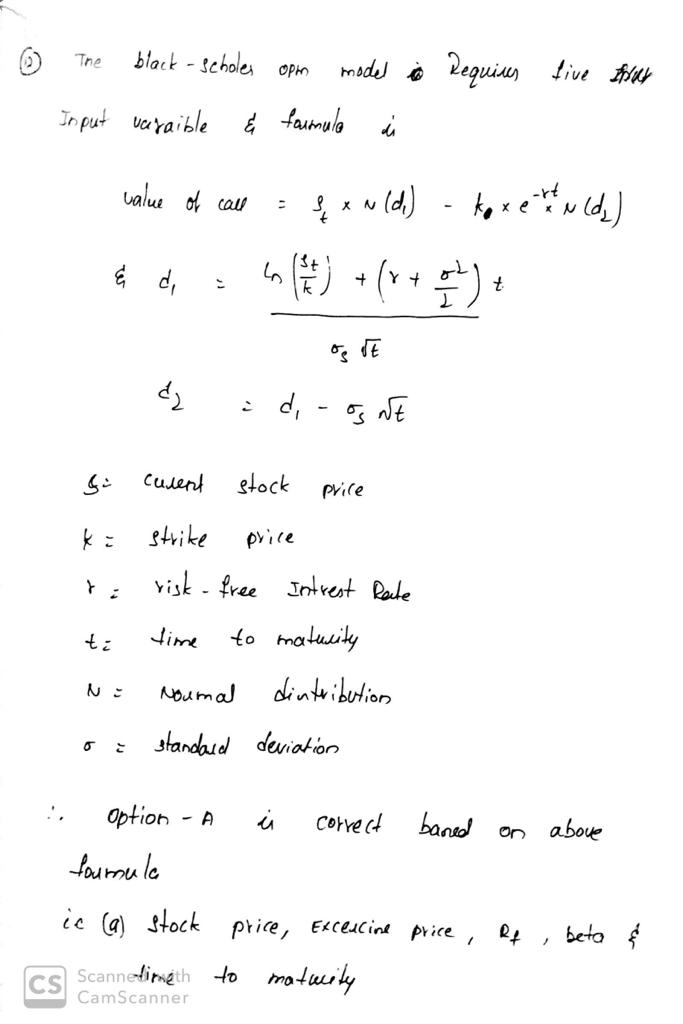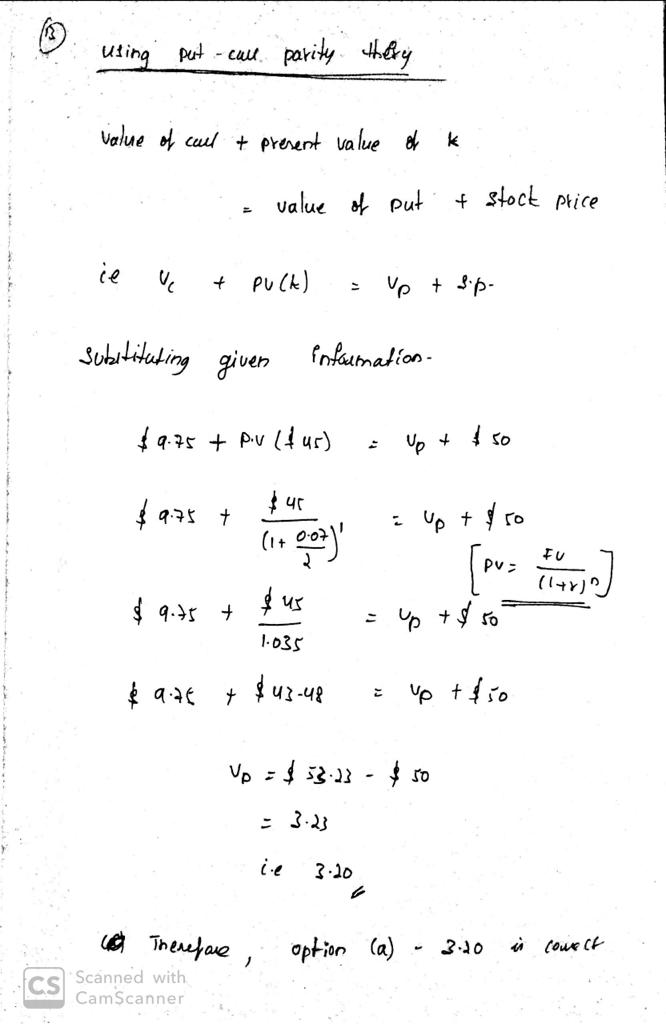Know the answer?
Your Answer:

#### Post as a guest

Your Name:

What's your source?

#### Earn Coin

Coins can be redeemed for fabulous gifts.

Not the answer you're looking for? Ask your own homework help question. Our experts will answer your question WITHIN MINUTES for Free.
Similar Homework Help Questions
• ### 2. (a) (5 points) State the Black-Scholes formulas for the prices at time 0 of a European call and put options on a non-dividend-paying stock ABC (b) (10 points) Consider an option on a non-divid...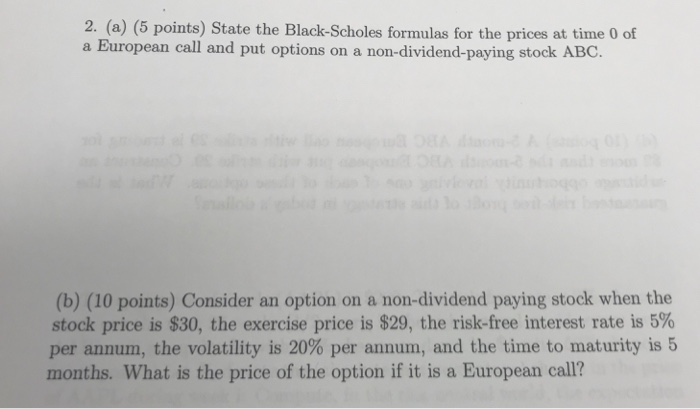2. (a) (5 points) State the Black-Scholes formulas for the prices at time 0 of a European call and put options on a non-dividend-paying stock ABC (b) (10 points) Consider an option on a non-dividend paying stock when the stock price is \$30, the exercise price is \$29, the risk-free interest rate is 5% per annum, the volatility is 20% per annum, and the time to maturity is 5 months. What is the price of the option if it is...

• ### Consider an option on a non-dividend-paying stock when the stock price is \$30, the exercise price...

Consider an option on a non-dividend-paying stock when the stock price is \$30, the exercise price is \$29, the risk-free interest rate is 5% per annum, the volatility is 25% per annum, and the time to maturity is four months. Use the Black-Scholes-Merton formula. What is the price of the option if it is a European call? What is the price of the option if it is an American call? What is the price of the option if it is...

• ### QUESTION # 10 Consider an option on a non-dividend paying stock when the stock price is \$90, the exercise price is \$98 the risk-free rate is 7% per annum, the volatility is 49% per annum, and the...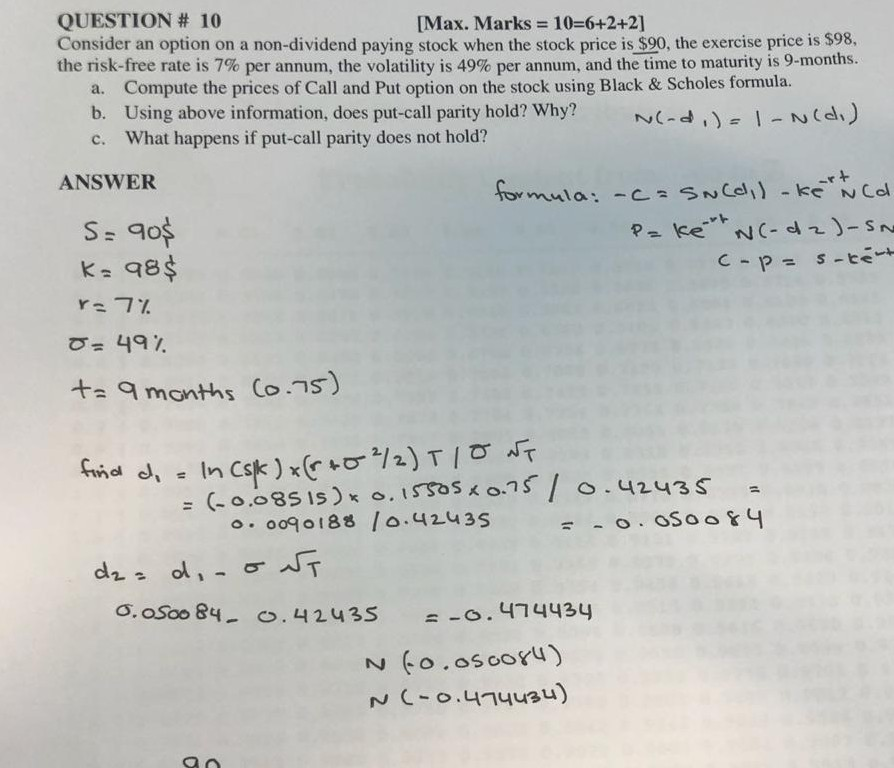QUESTION # 10 Consider an option on a non-dividend paying stock when the stock price is \$90, the exercise price is \$98 the risk-free rate is 7% per annum, the volatility is 49% per annum, and the time to maturity is 9-months. a. Compute the prices of Call and Put option on the stock using Black & Scholes formula. b. Using above information, does put-call parity hold? Why?-dNCa) c. What happens if put-call parity does not hold? [Max. Marks =...

• ### 6) Consider an option on a non-dividend paying stock when the stock price is \$38, the exercise price is \$40, the risk-free interest rate is 6% per annum, the volatility is 30% per annum, and the time...

6) Consider an option on a non-dividend paying stock when the stock price is \$38, the exercise price is \$40, the risk-free interest rate is 6% per annum, the volatility is 30% per annum, and the time to maturity is six months. Using Black-Scholes Model, calculating manually, a. What is the price of the option if it is a European call? b. What is the price of the option if it is a European put? c. Show that the put-call...

• ### Use Black Scholes to Value the put and call given the following criteria. The stock price...

Use Black Scholes to Value the put and call given the following criteria. The stock price six months from the expiration of an option is \$43.00, the exercise price of the option is \$39, the risk free interest rate is 10 percent per annum, and the volatility is 20% per annum.   A) c = 6.33, p = 0.43 B) c = 3.16, p = 1.06 C) c = 4.00, p = 1.90

• ### Evaluate and compute call and put options price for Star Ltd with reference to Black Scholes’...

Evaluate and compute call and put options price for Star Ltd with reference to Black Scholes’ option pricing model, with a dividend payout of \$ 2 in 30 days Star Ltd stock price                          = \$ 60.25 Exercise price                                    = \$ 50 Risk free rate                                     = 5.24%                Call maturity                                       = 270 days Stock volatility                                   = 0.45

• ### Consider the following call option: The current price of the stock on which the call option...

Consider the following call option: The current price of the stock on which the call option is written is \$32.00; The exercise or strike price of the call option is \$30.00; The maturity of the call option is .25 years; The (annualized) variance in the returns of the stock is .16; and The risk-free rate of interest is 4 percent. Use the Black-Scholes option pricing model to estimate the value of the call option.

• ### 5. Use the Black-Scholes methodology to find, by direct calculation, an explicit formula for the fair...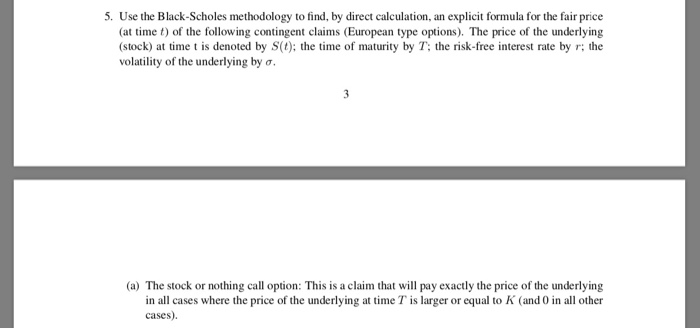5. Use the Black-Scholes methodology to find, by direct calculation, an explicit formula for the fair price (at time t) of the following contingent claims (European type options). The price of the underlying (stock) at time t is denoted by S(0); the time of maturity by T; the risk-free interest rate by r; the volatility of the underlying by o (a) The stock or nothing call option: This is a claim that will pay exactly the price of the underlying...

• ### Problem 21-12 Black–Scholes model Use the Black–Scholes formula to value the following options: a. A call...

Problem 21-12 Black–Scholes model Use the Black–Scholes formula to value the following options: a. A call option written on a stock selling for \$68 per share with a \$68 exercise price. The stock's standard deviation is 6% per month. The option matures in three months. The risk-free interest rate is 1.75% per month. (Do not round intermediate calculations. Round your answer to 2 decimal places.) b. A put option written on the same stock at the same time, with the...

• ### Please show every math step. An analyst is interested in using the Black-Scholes model to value...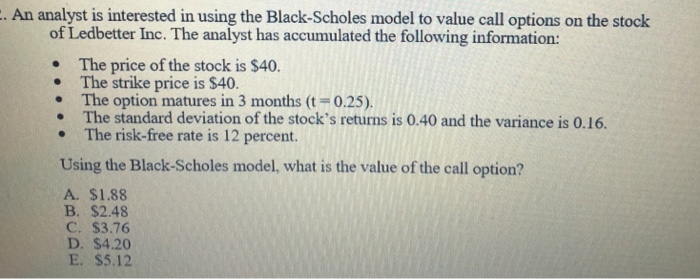Please show every math step. An analyst is interested in using the Black-Scholes model to value call options on the stock of Ledbetter Inc. The analyst has accumulated the following information: . The price of the stock is \$40 The strike price is \$40. . The option matures in 3 months (t 0.25) The standard deviation of the stock's returns is 0.40 and the variance is 0.16. The risk-free rate is 12 percent. Using the Black-Scholes model, what is the...

Free Homework App

Scan Your Homework
to Get Instant Free Answers
Need Online Homework Help?

Get Answers For Free
Most questions answered within 3 hours.